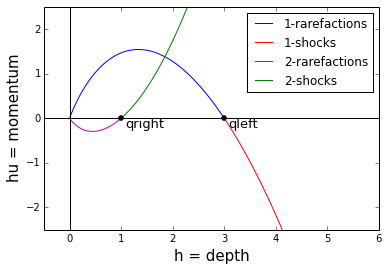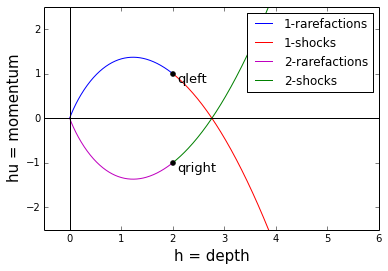# Solution of some Riemann problems for the shallow water equations¶

This notebook illustrates the solution to several Riemann problems. In each case an animation shows the evolution of the depth $h(x,t)$ along with a passive tracer (the color of the water) that helps visualize the fluid velocity. The solution always consists of two waves, each of which might be a shock wave or a rarefaction wave depending on where the left and right states lie in the phase plane. For each case, a phase plane plot is shown that illustrates how the intermediate state and the nature of each wave can be determined by looking at the intersection of Hugoniot loci and/or integral curves. This theory is discussed in Chapter 13 of the book "Finite Volume Methods for Hyperbolic Problems" and gives more dynamic version of some of the plots in that chapter, see also http://clawpack.github.io/doc/fvmbook.html.

This notebook can be found in the Clawpack apps repository, see http://clawpack.github.io/doc/apps.html for instructions on downloading this repository.

Note that if you download this notebook alone (e.g. from nbviewer), you should be able to run the code that produces the phase plane plots (self-contained in the cells below) but not the cells that run Clawpack (the cells starting with %%system) or the cells that produce animations from those results.

See http://clawpack.github.io/doc/notebooks.html for other Clawpack notebooks.

First set up various things needed for all the examples:

In :
%pylab inline

Populating the interactive namespace from numpy and matplotlib


Set the CLAW as environment variable before starting notebook, or specify as the second argument to os.environ.get below:

In :
import os, sys
CLAW = os.environ.get('CLAW','/Users/rjl/git/clawpack')
sys.path.append(CLAW)  # add to path so clawpack modules can be found
os.environ['CLAW'] = CLAW
os.environ['PYTHONPATH'] = CLAW  # so Fortran Makefiles work
print "Clawpack directory:  ",CLAW

Clawpack directory:   /Users/rjl/git/clawpack

In :
example_dir = CLAW + '/apps/notebooks/riemann/shallow'
os.chdir(example_dir)
print "Directory where this notebook is located:  ", os.getcwd()

Directory where this notebook is located:   /Users/rjl/git/clawpack/apps/notebooks/riemann/shallow

In :
%%system
make .exe  # Compile the Fortran code and produce executable

Out:
["make: Nothing to be done for .exe'."]
In :
import setrun
rundata = setrun.setrun()  # initialize most run-time variables for clawpack
outdir = '_output'


### For making the animations below:¶

In :
import glob
from matplotlib import image
from clawpack.visclaw.JSAnimation import IPython_display
from matplotlib import animation

def init():
return im,

def animate(i):
im.set_data(image_i)
return im,


### Set up scripts to plot solution in phase plane $hu$ vs. $h$:¶

In :
g = 1.  # gravitational constant used in book

def integral_curve(h, hstar, hustar, wave_family):
"""Return hu as a function of h for integral curves through (hstar, hustar)."""
ustar = hustar / hstar
if wave_family == 1:
hu = h*ustar + 2*h*(sqrt(g*hstar) - sqrt(g*h))
else:
hu = h*ustar - 2*h*(sqrt(g*hstar) - sqrt(g*h))
return hu

def hugoniot_locus(h, hstar, hustar, wave_family):
"""Return hu as a function of h for the Hugoniot locus through (hstar, hustar)."""
ustar = hustar / hstar
alpha = h - hstar
d = sqrt(g*hstar*(1 + alpha/hstar)*(1 + alpha/(2*hstar)))
if wave_family == 1:
hu = hustar + alpha*(ustar - d)
else:
hu = hustar + alpha*(ustar + d)
return hu

def phase_plane_curves(hstar, hustar, state, wave_family='both'):
"""
Plot the curves of points in the h - hu phase plane that can be connected to (hstar,hustar).
state = 'qleft' or 'qright' indicates whether the specified state is ql or qr.
wave_family = 1, 2, or 'both' indicates whether 1-waves or 2-waves should be plotted.
Colors in the plots indicate whether the states can be connected via a shock or rarefaction.
"""

h = linspace(0, hstar, 200)

if wave_family in [1,'both']:
if state == 'qleft':
hu = integral_curve(h, hstar, hustar, 1)
plot(h,hu,'b', label='1-rarefactions')
else:
hu = hugoniot_locus(h, hstar, hustar, 1)
plot(h,hu,'r', label='1-shocks')

if wave_family in [2,'both']:
if state == 'qleft':
hu = hugoniot_locus(h, hstar, hustar, 2)
plot(h,hu,'g', label='2-shocks')
else:
hu = integral_curve(h, hstar, hustar, 2)
plot(h,hu,'m', label='2-rarefactions')

h = linspace(hstar, 5, 200)

if wave_family in [1,'both']:
if state == 'qright':
hu = integral_curve(h, hstar, hustar, 1)
plot(h,hu,'b', label='1-rarefactions')
else:
hu = hugoniot_locus(h, hstar, hustar, 1)
plot(h,hu,'r', label='1-shocks')

if wave_family in [2,'both']:
if state == 'qright':
hu = hugoniot_locus(h, hstar, hustar, 2)
plot(h,hu,'g', label='2-shocks')
else:
hu = integral_curve(h, hstar, hustar, 2)
plot(h,hu,'m', label='2-rarefactions')

# plot and label the point (hstar, hustar)
plot([hstar],[hustar],'ko',markersize=5)
text(hstar + 0.1, hustar - 0.2, state, fontsize=13)

def make_axes_and_label(x1=-.5, x2=6., y1=-2.5, y2=2.5):
plot([x1,x2],[0,0],'k')
plot([0,0],[y1,y2],'k')
axis([x1,x2,y1,y2])
legend()
xlabel("h = depth",fontsize=15)
ylabel("hu = momentum",fontsize=15)


## Dam break Riemann problem:¶

The initial velocity is zero and the depth is greater to the left of $x=0$ than to the right. The solution is a shock wave moving to the right and a rarefaction wave moving to the left. The intermediate fluid velocity is positive.

In :
rundata.probdata.hl = hl = 3.
rundata.probdata.ul = ul = 0.
rundata.probdata.hr = hr = 1.
rundata.probdata.ur = ur = 0.
rundata.write()

In :
%%system
make output > output.txt
make plots > plots.txt

Out:
['python(36785,0x7fff75847310) malloc: *** error for object 0x100ba1810: pointer being freed was not allocated',
'*** set a breakpoint in malloc_error_break to debug',
'make: *** [plots] Abort trap: 6']
In :
figno = 1
fname = '_plots/*fig' + str(figno) + '.png'
filenames=sorted(glob.glob(fname))

fig = plt.figure(figsize=(10, 6),dpi=80)
ax = fig.add_axes([0, 0, 1, 1])
ax.axis('off')

animation.FuncAnimation(fig, animate, init_func=init,
frames=len(filenames), interval=200, blit=True)

Out:

Once Loop Reflect

### Phase Plane solution¶

The solution for the dam break problem consists of a 1-rarefaction and a 2-shock. In the phase plane, the intermediate state is where the 1-wave integral curve from $q_l = (h_l,h_lu_l)$ intersects the 2-wave Hugoniot locus from $q_r = (h_r,h_ru_r)$

In :
phase_plane_curves(hl, hl*ul, state='qleft', wave_family=1)
phase_plane_curves(hr, hr*ur, state='qright', wave_family=2)
make_axes_and_label()## Symmetric inflow results in two outgoing shock waves¶

The intermediate fluid velocity is zero, so this also flow models a wall at x=0 with flow towards wall:

In :
rundata.probdata.hl = hl = 2.
rundata.probdata.ul = ul = 0.5
rundata.probdata.hr = hr = 2.
rundata.probdata.ur = ur = -0.5
rundata.write()

In :
%%system
make output > output.txt
make plots > plots.txt

Out:
['python(36821,0x7fff75847310) malloc: *** error for object 0x10183aa10: pointer being freed was not allocated',
'*** set a breakpoint in malloc_error_break to debug',
'make: *** [plots] Abort trap: 6']
In :
fig = plt.figure(figsize=(10, 6),dpi=80)
ax = fig.add_axes([0, 0, 1, 1])
ax.axis('off')

animation.FuncAnimation(fig, animate, init_func=init,
frames=len(filenames), interval=200, blit=True)

Out:

Once Loop Reflect

### Phase Plane solution¶

The phase plane solution in this case consists of two shock waves and the intermediate state is where the 2-wave Hugoniot locus from $q_l = (h_l,h_lu_l)$ intersects the 1-wave Hugoniot locus from $q_r = (h_r,h_ru_r)$

In :
phase_plane_curves(hl, hl*ul, state='qleft', wave_family=1)
phase_plane_curves(hr, hr*ur, state='qright', wave_family=2)
make_axes_and_label()## Symmetric outflow giving double rarefaction wave (flow away from wall)¶

In :
rundata.probdata.hl = hl = 2.
rundata.probdata.ul = ul = -0.5
rundata.probdata.hr = hr = 2.
rundata.probdata.ur = ur = 0.5
rundata.write()

In :
%%system
make output > output.txt
make plots > plots.txt

Out:
['python(36852,0x7fff75847310) malloc: *** error for object 0x10687c410: pointer being freed was not allocated',
'*** set a breakpoint in malloc_error_break to debug',
'make: *** [plots] Abort trap: 6']
In :
fig = plt.figure(figsize=(10, 6),dpi=80)
ax = fig.add_axes([0, 0, 1, 1])
ax.axis('off')
`